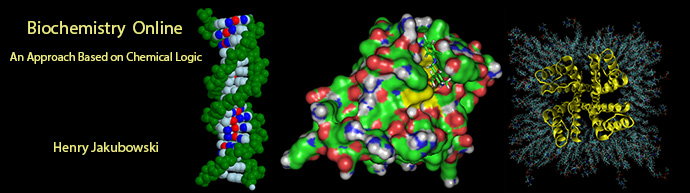# Biochemistry Online: An Approach Based on Chemical Logic# CHAPTER 5 - BINDING

## A:  INTRODUCTION TO REVERSIBLE BINDING

BIOCHEMISTRY - DR. JAKUBOWSKI

Last Update:  3/25/16

•  Learning Goals/Objectives for Chapter 5A:  After class and this reading, students will be able to write equations for the dissociation constant (KD), mass balance of total macromolecule (M0), total ligand (L0), and [ML]  as a function of L or Lo ([ML] = [M0][L]/(KD+ [L])  (when Lo >> Mo or when free [L] is known) and Y = fractional saturation =  Y = ([ML]/[M0] = [L]/(KD+ [L]) decide which of two given equations for [ML] should be used under conditions when the above conditions for L0 and L are given based on the equation ([ML] = [M0][L]/(KD+ [L]) draw qualitative graphs for different given L0, L, and Kd values determine fraction saturation given relatives values of Kd and L, assuming L0 >> M0 compare relative % bound for covalent binding of protons to an acid and noncovalent binding of a ligand to a macromolecule given pka/pH and Kd/L values describe differences in binding curves for binding of a ligand to a macromolecule and the dimerization of a macromolecule derive an equation which shows the relationships between the rate constant for binding (kon), dissociation (koff) and the thermodynamic dissociation (Kd) or equilibrium constant (Keq). describe the structural and mathematic differences between specific and nonspecific binding given a Kd, estimate t1/2 values for the lifetime of the ML complex. describe techniques used to determine ML for given L or L0 values, including those that do and do not require separation of ML from M , so that Kd values for a M and L interaction can be determined List advantages of isothermal titration calorimetry and surface plasmon resonance in determination of binding interaction parametersNavigation

Archived version of full Chapter 5A:  Introduction to Reversible Binding

•